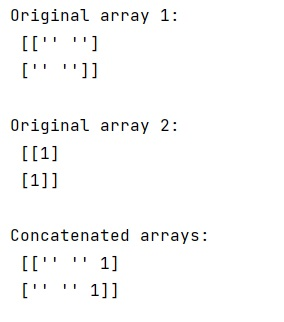# How to store different datatypes in one NumPy array?

Learn, how to store different datatypes in one NumPy array in Python?
Submitted by Pranit Sharma, on February 08, 2023

NumPy is an abbreviated form of Numerical Python. It is used for different types of scientific operations in python. Numpy is a vast library in python which is used for almost every kind of scientific or mathematical operation. It is itself an array which is a collection of various methods and functions for processing the arrays.

## Storing different datatypes in one NumPy array

In a programming language, data types are the particular format in which a value is being stored. A data type is a kind of data item, which represents what values it can take, the programming language used, or the operations that can be performed on it.

Suppose that we are given two NumPy arrays, one with strings and the other with ints. We need to concatenate these numpy arrays so that we can store both types of values in one single NumPy array.

For this purpose, we will create an array of data type string and others with data type int and we will use the hstack() method to concatenate them.

Let us understand with the help of an example,

## Python code to store different datatypes in one NumPy array

```# Import numpy
import numpy as np

# Creating two numpy arrays
a1 = np.zeros((2,2), dtype='U2')
a2 = np.ones((2,1), dtype='O')

# Display original arrays
print("Original array 1:\n",a1,"\n")
print("Original array 2:\n",a2,"\n")

# Concatenating arrays
res = np.hstack([a1,a2])

# Display the result
print("Concatenated arrays:\n",res,"\n")
```

Output: